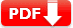# GED Math Practice Test with Answers 2021 Printable PDF

Download GED Math Practice Test with Answers 2021 Printable PDF: Our GED Sample Questions Answers available in Free Printable PDF and Editable Doc files. This math test Topics Basic Math, Geometry, Basic Algebra, Graphs, and Functions. Please note: this is not an actual question. Our practice test is just for testing your knowledge and will help you for GED Test Prep online.

 Test Name GED Practice Test GED full form General Educational Development Subject Name Mathematics Mode of Exam Computer-based adaptive test Test Type Math Sample / Mock Test 3 Available Printable PDF YES (Download link is given below) Total Question (MCQs) 35 Available of Answers YES Explanation YES Topics Basic Math Geometry Basic Algebra Graphs and Functions

## GED Math Practice Test with Answers 2020

Q1. The dimensions of Box B, shown below, are twice the length of the corresponding dimensions on Box A (not shown).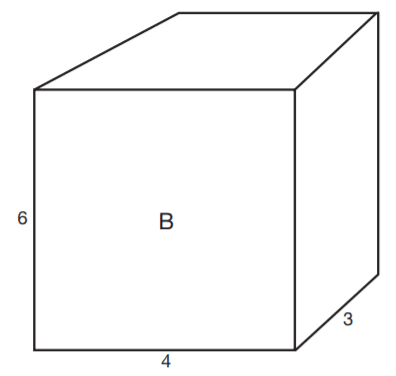• A). The volume of Box A is greater than the volume of Box B.
• B). The volume of Box B is twice the volume of Box A.
• C). The volume of Box B is three times the volume of Box A.
• D). The volume of Box B is four times the volume of Box A.
• E). The volume of Box B is eight times the volume of Box A.

Since the dimensions of Box A are half of the dimensions of Box B, the side lengths must be 3, 2, and 1.5. Next, find the volumes of the two
boxes. Use the formula V = lwh. The volume of Box B is 72, and the volume of Box A is 9. 72 is 8 times larger than 9.

Q2. At the end of baseball season, 5% of the children enrolled in a local youth baseball program will be chosen to play in the state tournament. If 12 children will be chosen to play in the tournament, how many children are enrolled in the
program?

• A). 24
• B). 60
• C). 120
• D). 240
• E). 600

Set up a proportion: 5/100 = 12/x , where x is the number of children enrolled in the program. 12 × 100 = 1,200, and 1,200 ÷ 5 = 240.

Q3. A bag contains 12 red, 3 blue, 6 green, and 4 yellow marbles. If a marble is drawn from the bag at random, what is the probability that the marble will be either blue or yellow?

• A). 7%
• B). 12%
• C). 16%
• D). 25%
• E). 28%

Add the number of marbles to get the total number in the bag; 12 + 3 + 6 + 4 = 25. Therefore, 25 is the number of possible outcomes.
Seven marbles are either blue or yellow. Seven is the number of favorable outcomes (7/25) x (4/4) =28/100 =28%

Q4. Patricia wants to order business cards. A printing company determines the cost (C) to the customer using the following function, where b = the number of boxes of cards and n = number of ink colors.
C = $25.60b +$14.00b(n − 1) If Patricia orders 4 boxes of cards printed in 3 colors, how much will the cards cost?

• A). $214.40 • B).$168.00
• C). $144.40 • D).$102.40
• E). $56.00 View Correct Answer Answer: A Substitute 4 for b and 3 for n into the function. Then, solve the equation. C =$25.60(4) + $14(4)(3 − 1) =$102.40 + $112.00 =$214.40

Q5. Andrea bought a used mountain bike for $250. She gave the bike a new paint job; replaced the tires, chain, and gear assembly; and sold the bike for 150% of the price she paid. For what amount, in dollars, did she sell the bike? • (You may have to enter the correct value) View Correct Answer Answer: 375 Multiply$250 by 1.5, which equals $375. Q6. Ajani finds that the distance between two landmarks on a map is 6 (½) inches. If the map scale reads ¾ inch = 120 miles, what is the actual distance, in miles, between the two landmarks? • (You may have to enter the correct value) View Correct Answer Answer: 1040 Set up the proportion and solve: x = the number of miles between the two landmarks. Q7. Which of the following is a graph of the inequality −2 ≤ x < 4?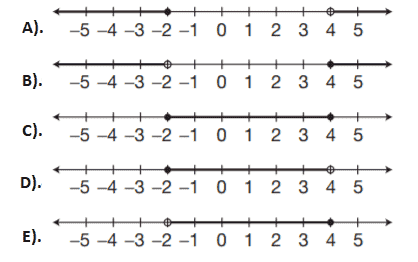View Correct Answer Answer: D Separate the two inequalities into two inequalities, x < 4 and −2 = x. Choice d is the only graph that represents the inequalities. There must be an open circle to represent that the 4 is not included and a shaded circle to represent that the −2 is included. Questions 8 and 9 are based on the following figure.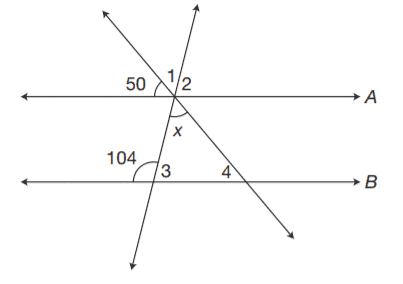Lines A and B are parallel. Q8. What is the measure of ∠4? • A). 50° • B). 76° • C). 126° • D). 130° • E). 104° View Correct Answer Answer: A Angle 4 and the angle measuring 50° are corresponding angles. Therefore,m∠4 = 50°. Q9. What is the value of x? • A). 54° • B). 76° • C). 126° • D). 130° • E). Not enough information is given View Correct Answer Answer: A Angle 3 and the angle measuring 104° are supplementary; therefore,m∠3 = 180 − 104 = 76. As established in the previous problem, angle 4 and the angle measuring 50° are corresponding angles, so m∠4 = 50°. Angle 3, angle 4, and x are the interior angles of a triangle, so they equal 180°; 50 + 76 + x = 180, and so x = 54°. Q10. On a coordinate plane, a vertical line is drawn through the point (−3,4). On the same plane, a horizontal line is drawn through the point (2,−1). At what point on the plane will the two lines intersect?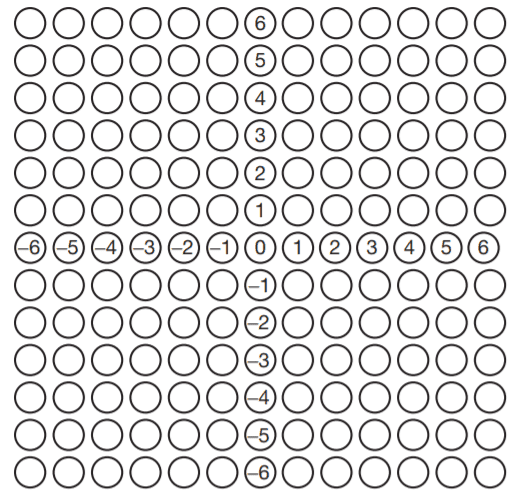• You may have to answer on the coordinate grid (screen) View Correct Answer Answer: (−3, −1). The vertical line is parallel to the y-axis, and all of its points have the x-coordinate −3. The horizontal line is parallel to the x-axis, and all of its points have the y-coordinate −1. Therefore, the coordinates are −3 and −1. Q11. What was Edmundo’s mean score for a round of golf in August if his scores for each round were 78, 86, 82, 81, 82, and 77? • A). 77 • B). 78 • C). 81 • D). 81 • E). 84 View Correct Answer Answer: C Mean = average. Add the scores and divide by the number of scores. 78 + 86 + 82 + 81 + 82 + 77 = 486 => 486 ÷ 6 = 81 Q12. In quadrilateral ABCD, side AB is parallel to side CD. Sides AD and BC are not parallel. What is the area of the figure to the nearest square centimeter?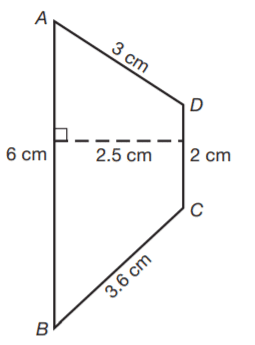• A). 10 cm² • B). 12 cm² • C). 14 cm² • D). 18 cm² • E). 20 cm² View Correct Answer Answer: A Quadrilateral ABCD is a trapezoid because it has one pair of parallel sides. The bases are the parallel sides, AB and CD. The height is 2.5 cm. Use the formula for the area of a trapezoid. Q13. A display of canned yams has a sign that reads “5 cans for$3.” At the same rate, how much would the store charge, to the nearest whole cent, for 8 cans?

• A).  1.80
• B).  2.80
• C).  3.80
• D).  4.80
• E).  8.80

Set up the proportion 5/3 = 8/x , where x is the cost of 8 cans of yams; 3 × 8 = 24, and 24 ÷ 5 = $4.80. Q14. A plastic pipe, 5 feet 9 inches long, is cut into three equal pieces. Assuming no waste when the cuts are made, what is the length of each piece? • A). 1 ft. 6 in. • B). 1 ft. 8 in. • C). 1 ft. 9 in. • D). 1 ft. 11 in. • E). 2 ft. View Correct Answer Answer: D 5 ft. 9 in. = 69 inches. Divide by 3: 69 ÷ 3 = 23 inches. Convert feet to inches, 23 in. = 1 ft. 11 in. Q15. The Wrights plan to carpet their bedroom and the adjoining hallway as shown in the diagram. What is the area of the bedroom and hallway in square feet?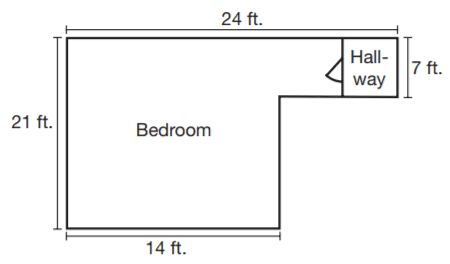• A). 147 • B). 336 • C). 294 • D). 364 • E). 504 View Correct Answer Answer: D Think of the space as two rectangles: 21 ft. by 14 ft. and 10 ft. by 7 ft. (You can find the length of the missing side of the smaller rectangle by subtracting: 24 − 14 = 10.) Use the formula A = lw to find the area of each rectangle, and then combine to find the total area of the floor to be carpeted: 21 × 14 = 294, and 7 × 10 = 70. Add the areas of the two rectangles: 294 + 70 = 364, so the Wrights will need to buy 364 square feet of carpeting. Q16. One number is 12 more than 3 times another number. The sum of the 2 numbers is −20. What are the numbers? • A). −2 and −18 • B). −4 and −16 • C). −5 and −15 • D). −6 and −14 • E). −8 and −12 View Correct Answer Answer: E x = one number and 3x + 12 = the other number, for the equation: x + 3x + 12 = − 20 4x + 12 = −20 4x = −32 x = −8 3(−8) + 12 = −12 Questions 17 and 18 refer to the following graph.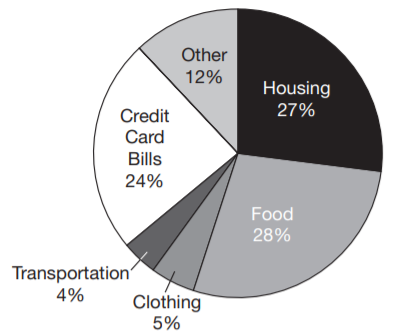The Kleins are trying to pay off their credit card debt, so they developed the following budget based on their monthly take-home pay. Q17. If the Kleins’ monthly take-home pay is$2,500, about how much do they plan to pay each month on their credit card debt?

• A). $600 • B).$450
• C). $300 • D).$240
• E). Not enough information is given.

Find 24% of $2,500. Q18. What fraction of the Kleins’ monthly take-home pay goes toward clothing? • A). 1/20 • B). 3/20 • C). 1/40 • D). 2/40 • E). 3/50 View Correct Answer Answer: A Clothing expenses take 5% of the Wrights’ pay. Change 5% to a fraction to get 5/100, which can also be reduced to 1/20. Q19. The graph of the equation y = − (3/4) x + 1 is a line that passes through points C and D on the coordinate plane. Which of the following points also lies on the graph of the equation? • A). (2,0) • B). (3,−1) • C). (5,−3) • D). (8,−5) • E). . (10,−6) View Correct Answer Answer: D Either locate each point on the grid and compare it to the line or substitute the x and y values from each ordered pair into the equation: y = − ¾ x + 1 −5 = − ¾ (8) + 1 −5 = −6 + 1 −5 = −5 Q20. The sum of three consecutive even integers is 90. What is the greatest number in the series? • A). 26 • B). 28 • C). 30 • D). 32 • E). 34 View Correct Answer Answer: D The three numbers can be represented by x, x + 2, and x + 4. Solve the equation: x + x + 2 + x + 4 = 90 3x + 6 = 90 3x = 84 x = 28 The three numbers are 28, 30, 32. The question asks for the largest of these. Q21. The three interior angles of ΔJKL measure 45°, 45°, and 90°. The three interior angles of ΔPQR measure 45°, 45°, and 90°. The side JK of JKL measures 4 cm. Based on the given information, which of the following must be a true statement? • A). Δ JKL and ΔPQR are acute triangles. • B). One side of ΔPQR measures 4 cm • C). The perimeter of ΔJKL is 12 cm. • D). ΔJKL and ΔPQR are congruent triangles. • E). ΔJKL and ΔPQR are similar triangles. View Correct Answer Answer: E Similar triangles have the same angle measures. Congruent triangles have the same angle measures and the same side lengths. From the given information, you cannot know if the side lengths are the same, so you can conclude only that the triangles are similar. Q22. A parallelogram is drawn on a coordinate grid so that three vertices are located at (3,4), (−2,4), and (−4,1). At what coordinates should the fourth vertex be located?View Correct Answer Answer: (1,1). Plot the points given in the problem and compete with the parallelogram. Remember that in a parallelogram, both pairs of opposite sides are equal and parallel. Q23. Eight athletes ran a 1 mile race. The winner’s time was 4 minutes 8 seconds. If the median time was 4 minutes 48 seconds, what was the time of the athlete who finished last? • A). 5 min. 28 sec. • B). 5 min. 4 sec. • C). 4 min. 46 sec. • D). 4 min. 28 sec. • E). Not enough information is given. View Correct Answer Answer: E The median time is the middle time. There is no way of knowing how far behind the median the slowest runner was. Questions 24 and 25 refer to the following information.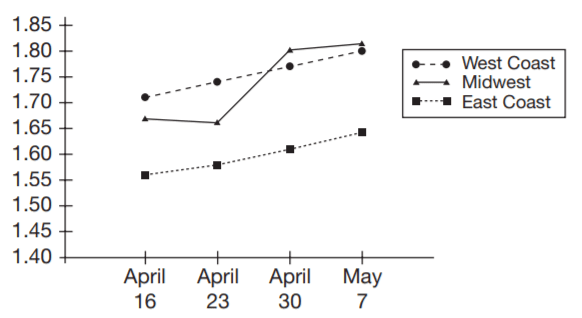Q24. On what date and in what location was there the greatest jump in the price of gasoline from one week to the next? • A). April 23 on the West Coast • B). April 30 in the Midwest • C). April 30 on the West Coast • D). May 7 on the East Coast • E). May 7 in the Midwest View Correct Answer Answer: B The steepest rise on the graph was from April 23 to April 30. The symbol indicates that it was in the Midwest. Q25. Based on the information in the graph, which of the following is the best prediction of the price per gallon of gasoline on the West Coast for the week following May 7? • A).$1.64
• B). $1.71 • C).$1.76
• D). $1.82 • E).$1.86

The prices for the West Coast have been rising steadily by 2 or 3 cents each week. On May 7, the price on the West Coast is a little beneath $1.80. If it rises 2 or 3 cents, it should be at about$1.82 by the following week. The question gives no reason to expect a sudden decline in price or a sharp increase.

Q26. Chikita made three long-distance calls. According to her phone bill, the calls were 19 minutes, 24 minutes, and 8 minutes in length. If Chikita pays 9 cents per minute on all long-distance calls, how much was she billed for the three calls?

• A). $2.70 • B).$4.59
• C). $5.10 • D).$13.77
• E). $15.30 View Correct Answer Answer: B Add the times and multiply by 9 cents: 19 + 24 + 8 = 51 minutes; 51 × .09 =$4.59.

Q27. Maggie and Christian decided to share the cost of buying their friends a wedding gift. Maggie put in $20 less than twice the amount that Christian contributed. Together, they spent$94. How  many dollars did Maggie contribute toward the gift?

• A). $56 • B).$76
• C). $86 • D).$96
• E). $106 View Correct Answer Answer: A Let x represents the amount that Christian put in and 2x − 20 represents Maggie’s contribution. Solve the equation. x + 2x − 20 = 94 3x = 114 x = 38 Christian put in$38 and Maggie put in 94 − 38 = \$56.

Q28. The Northridge Quakers have won 20 games and lost 15. What is the ratio of games won to game played?

• A). 3:4
• B). 3:7
• C). 4:3
• D). 4:7
• E). 4:10

The number of games played is the total of the wins and losses, (20 + 15 = 35). Write the ratio and simplify. 20/35 = 4/7

Q29. To set up a tent, workers place a 25-foot pole in the center of a grassy area as shown in the diagram. A bracing wire is attached to the top of the pole and to a stake 9 feet from the base of the pole. Which of the following represents the
length of the bracing wire?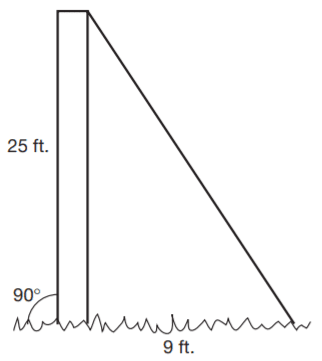• A). √1156
• B). √706
• C). √625
• D). √256
• E). √34
Use the Pythagorean theorem 25² + 9² = c²:

Q30. What is the value of the expression −3 × 5² + 2(4 − 18) + 3²?

• A). −130
• B). −76
• C). −20
• D). 74
• E). 130

−3 × 5² + 2(4 − 18) + 3³
−3 × 25 + 2(−14) + 27
−75 + (−28) + 27
−76

Q31. The lengths of the sides of ΔABC are 6 inches, 8 inches, and 10 inches. Which of the following conclusions must be true?

• A). ∠C is a right angle
• B). ΔABC is an acute triangle.
• C). ΔABC contains one obtuse angle.
• D). ∠A is an acute angle.
• E). m∠A + m∠B + m∠C = 180°

ABC is a right triangle, but you have no way of knowing which angle is a right angle, so eliminate choice a. Regardless of the type of triangle,
the sum of the measure of the interior angles of the triangle must be 180°.

Q32. Two sides of a triangle measure 6 and 10 inches. If the triangle is a right triangle, which of the following could be the measure, in inches, of the third side?

• A). 4
• B). 6
• C). 8
• D). 10
• E). 12

You can try each combination of sides in the Pythagorean theorem. Only 6, 8, and 10 will work. 62 + 82 = 102, 36 + 64 = 100, 100 = 100.

Q33. Classify the figure containing interior angles 3, 6, 11, and 13.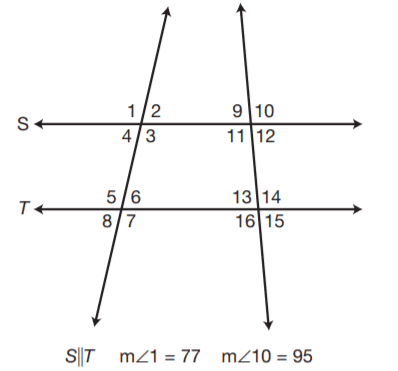• A). scalene triangle
• B). trapezoid
• C). parallelogram
• D). rectangle
• E). square

A four-sided figure with only one pair of parallel sides is a trapezoid.

Q34. The floor of a walk-in closet measures 7 feet by 4 feet. If the ceiling height is 8 feet, what is the volume in cubic feet of the closet?

• A). 28
• B). 56
• C). 112
• D). 168
• E). 224

A closet is the shape of a rectangular solid. To find the volume, multiply. V = lwh. 7(4)(8) = 224 cubic feet.

Q35. In a right triangle, the hypotenuse measures 15 inches. If one leg of the triangle measures 6 inches, which of the following equations could be used to find the length of the other leg (x) in inches?

• A). x = 15 + 6
• B). x = 15 − 6
• C). x = 15 − 6
• D). x² = 15² + 6²
• E). x² = 15² − 6²
 Document Type Download Link Free Editable Doc File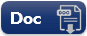Free Printable PDF File